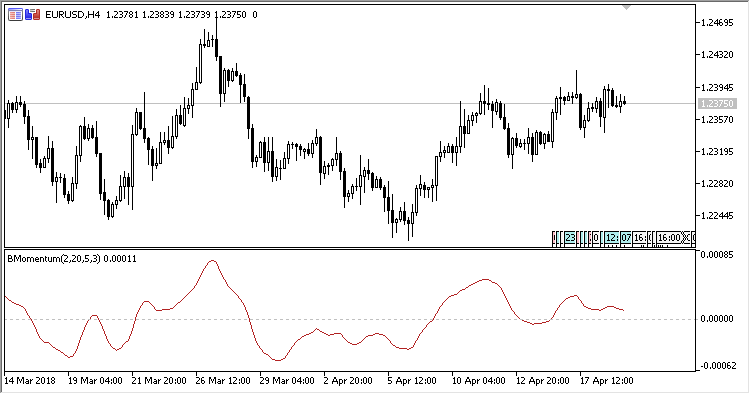# Blau_Mtm – indicator for MetaTrader 5

• A+
category：MT5 INDICATORS

The William Blau Momentum Oscillator shows the William blau momentum.

It has five input parameters:

• Period - calculation period;
• First smoothing period - the initial smoothin period;
• Second smoothing period - the secondary smoothing period;
• Third smoothing period - the resulting smoothing period;
• Applied price - price used for calculations.

Calculations:

`Blau_Momentum[i] = EMA(EMA(EMA(Mtm, Smooth period 1), Smooth period 2), Smooth period 3)`

where:

`Mtm[i] = Price[i] - Price[i-Period+1]`